Skip to content
Related Articles
Drop Empty Columns in Pandas
• Last Updated : 23 Dec, 2020

In this article, we will try to see different ways of removing the Empty column, Null column, and zeros value column. First, We will create a sample data frame and then we will perform our operations in subsequent examples by the end you will get a strong hand knowledge on how to handle this situation with pandas.

Approach:

• Import required python library.
• Create a sample Data Frame.
• Use the Pandas dropna() method, It allows the user to analyze and drop Rows/Columns with Null values in different ways.
• Display updated Data Frame.

Syntax: DataFrameName.dropna(axis=0, how=’any’, inplace=False)

Parameters:

• axis: axis takes int or string value for rows/columns. Input can be 0 or 1 for Integer and ‘index’ or ‘columns’ for String.
• how: how takes string value of two kinds only (‘any’ or ‘all’). ‘any’ drops the row/column if ANY value is Null and ‘all’ drops only if ALL values are null.
• inplace: It is a boolean which makes the changes in the data frame itself if True.

Sample Data:

This is the sample data frame on which we will perform different operations.

## Python3

 `# import required libraries``import` `numpy as np``import` `pandas as pd`` ` `# create a Dataframe``Mydataframe ``=` `pd.DataFrame({``'FirstName'``: [``'Vipul'``, ``'Ashish'``, ``'Milan'``],``                            ``"Gender"``: ["``", "``", "``"],``                            ``"Age"``: [``0``, ``0``, ``0``]})``Mydataframe[``'Department'``] ``=` `np.nan`` ` `# show the dataframe``print``(Mydataframe)`

Output: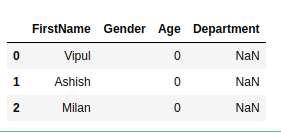Example 1:

Remove all null value column.

## Python3

 `# import required libraries``import` `numpy as np``import` `pandas as pd`` ` `# create a Dataframe``Mydataframe ``=` `pd.DataFrame({``'FirstName'``: [``'Vipul'``, ``'Ashish'``, ``'Milan'``],``                            ``"Gender"``: ["``", "``", "``"],``                            ``"Age"``: [``0``, ``0``, ``0``]})`` ` `Mydataframe[``'Department'``] ``=` `np.nan`` ` `display(Mydataframe)`` ` `Mydataframe.dropna(how``=``'all'``, axis``=``1``, inplace``=``True``)`` ` `# show the dataframe``display(Mydataframe)`

Output: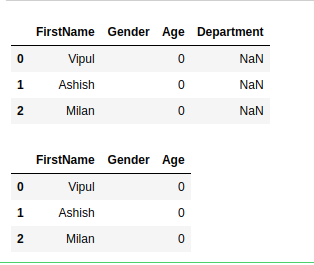Example 2:

Replace all Empty places with null and then Remove all null values column with dropna function.

## Python3

 `# import required libraries``import` `numpy as np``import` `pandas as pd`` ` `# create a Dataframe``Mydataframe ``=` `pd.DataFrame({``'FirstName'``: [``'Vipul'``, ``'Ashish'``, ``'Milan'``],``                            ``"Gender"``: ["``", "``", "``"],``                            ``"Age"``: [``0``, ``0``, ``0``]})`` ` `Mydataframe[``'Department'``] ``=` `np.nan``display(Mydataframe)`` ` `nan_value ``=` `float``(``"NaN"``)``Mydataframe.replace("", nan_value, inplace``=``True``)`` ` `Mydataframe.dropna(how``=``'all'``, axis``=``1``, inplace``=``True``)`` ` `# show the dataframe``display(Mydataframe)`

Output: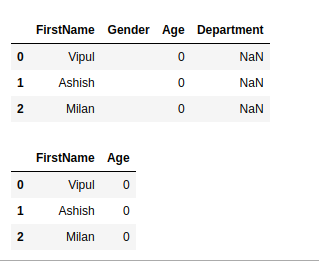Example 3:

Replace all zeros places with null and then Remove all null values column with dropna function.

## Python3

 `# import required libraries``import` `numpy as np``import` `pandas as pd`` ` `# create a Dataframe``Mydataframe ``=` `pd.DataFrame({``'FirstName'``: [``'Vipul'``, ``'Ashish'``, ``'Milan'``],``                            ``"Gender"``: ["``", "``", "``"],``                            ``"Age"``: [``0``, ``0``, ``0``]})`` ` `Mydataframe[``'Department'``] ``=` `np.nan``display(Mydataframe)`` ` `nan_value ``=` `float``(``"NaN"``)``Mydataframe.replace(``0``, nan_value, inplace``=``True``)`` ` `Mydataframe.dropna(how``=``'all'``, axis``=``1``, inplace``=``True``)`` ` `# show the dataframe``display(Mydataframe)`

Output: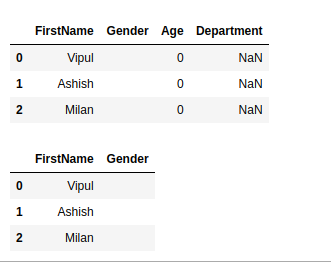Example 4:

Replace all zeros and empty places with null and then Remove all null values column with dropna function.

## Python3

 `# import required libraries``import` `numpy as np``import` `pandas as pd`` ` `# create a Dataframe``Mydataframe ``=` `pd.DataFrame({``'FirstName'``: [``'Vipul'``, ``'Ashish'``, ``'Milan'``],``                            ``"Gender"``: ["``", "``", "``"],``                            ``"Age"``: [``0``, ``0``, ``0``]})`` ` `Mydataframe[``'Department'``] ``=` `np.nan``display(Mydataframe)`` ` `nan_value ``=` `float``(``"NaN"``)``Mydataframe.replace(``0``, nan_value, inplace``=``True``)``Mydataframe.replace("", nan_value, inplace``=``True``)`` ` `Mydataframe.dropna(how``=``'all'``, axis``=``1``, inplace``=``True``)`` ` `# show the dataframe``display(Mydataframe)`

Output: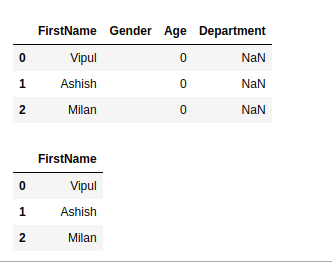Attention geek! Strengthen your foundations with the Python Programming Foundation Course and learn the basics.

To begin with, your interview preparations Enhance your Data Structures concepts with the Python DS Course. And to begin with your Machine Learning Journey, join the Machine Learning – Basic Level Course

My Personal Notes arrow_drop_up